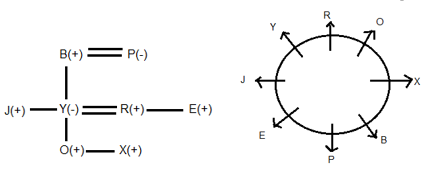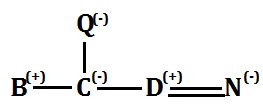Latest Banking jobs   »   Reasoning Ability Quiz for IBPS 2020...

# Reasoning Ability Quiz for IBPS 2020 Mains Exams- 23rd December

Directions (1-5): Study the following information carefully and answer the question given below-
In a family, there are eight members i.e. R, E, X, B, Y, J, P and O who are sitting around a circular table and facing to opposite to centre. There are three generation in which two married couple and only two female members are there. B is maternal grandfather of O and sits immediate right of X. The daughter of B sits opposite to B. R is son- in law of P who is mother of J. E is brother of R who is father of O. E is paternal uncle of X who is grandson of P. The brother of Y is J who is unmarried. J is maternal Uncle of O and sits immediate left of Y. Y is mother of X and B has only two children. R is an immediate neighbor of Y. P does not sit adjacent to her any grandson. E sits 2nd right of B and he is unmarried.
Q1. Who is the father of X?
(a) P
(b) B
(c) R
(d) J
(e) E

Q2. Who among the following sits 2nd left of Y’s mother?
(a) X
(b) B
(c) R
(d) J
(e) E

Q3. Who among the following sits opposite to O?
(a) P
(b) B
(c) R
(d) J
(e) E

Q4. How many members sit between R and E when counted left to R?
(a) One
(b) Two
(c) Three
(d) Four
(e) None

Q5. Who among the following is father of J?
(a) O
(b) X
(c) E
(d) R
(e) B

Directions (6-10): In these questions the symbols #, ©, it, \$ and % are used with different meanings as given below:
‘A# B’ means ‘A is smaller than B’
‘A © B’ means ‘A is greater than B’
‘A π B’ means ‘A is either smaller than or equal to B’
‘A \$ B’ means ‘A is either greater than or equal to B’
‘A % B’ means ‘A is neither smaller nor greater than B’
In each of the following questions, assuming the given statements to be true, find out which of the two conclusions I and II given below them is/are definitely true. Give answer
(a) if only conclusion I is true.
(b) if only conclusion II is true.
(c) if either conclusion I or II is true.
(d) if neither conclusion I nor II is true.
(e) if both conclusions I and II are true.

Q6.Statement: L \$ M % Q © S # T π P
Conclusions:
II. P π Q

Q7. Statement: W # H π I % C \$ H © S
Conclusions:
I. W#S
II. C\$W

Q8. Statement: W % X © Y π Z © R % L
Conclusions:
II. X π R

Q9. Statement: A # N % O π T π H # E
Conclusions:
I. H % O

Q10. Statement: P © V % T # D # M \$ F
Conclusions:

Directions (11-15): Each of the questions below consists of a question and two statements numbered I and II given below it. You have to decide whether the data provided in the statements are sufficient to answer the question.
(a) If statements I alone is sufficient to answer the question, but statement II alone is not sufficient to answer the question.
(b) If statement II alone is sufficient to answer the question, but statement I alone is not sufficient to answer the question.
(c) If statement either I or II is sufficient to answer the question.
(d) If both the statements I and II taken together are not sufficient to answer the questions.
(e) If both the statements I and II taken together are sufficient to answer the questions.

Q11. What is Sonali’s position from the left end of a row?
I. There are 4 students between Gauri and Sonali. Bhavna is 6th to the right of Gauri.
II. Gini is 6th to the left of Sonali and is 2nd from the left end.

Q12. How many students are sitting between P and Q?
I. P is 5th to the right of R and 6th to the left of S. Q is 6th to the right of R.
II. In a row of 25 students, P is 5th from left end and Q is 20th from the right end.

Q13. Among G, H, I, J and K who among the following got third highest marks?
I. G has got 1 mark less than H and has got more marks than K.
II. I and J have got more marks than G.

Q14. How is N related to Q?
I. C is daughter of Q, who is also the mother of D.
II. B is brother of C and N is the wife of D.

Q15.Who has got highest marks among A, B, C, D and E?
I. D has got greater marks than A and less than C. Also, B has got greater marks than A and less than E.
II. E has got less marks than C and greater than B and D. A has got the lowest marks.

Practice More Questions of Reasoning for Competitive Exams:

###### Prelims Quiz Study Plan for SBI & IBPS Exams 2020

Solutions

Solutions (1-5):
Sol.S1.Ans.(c)
S2.Ans.(a)
S3.Ans.(e)
S4.Ans.(b)
S5.Ans.(e)

Solutions (6-10):
S6. Ans.(c)
S7. Ans.(d)
S8. Ans.(c)
S9. Ans.(c)
S10. Ans.(e)

Solutions (11-15):
S11. Ans.(b)
Sol. If the data in statement II alone is sufficient to answer the question.
From I: It is given that Gauri is 5th to Sonali but not given left or right
From II: Gini is 2nd from left end, and then Sonali is 6th to right of Gini, so Sonali is 8th from left end.

S12. Ans.(c)
Sol. If the data either in statement I alone or statement II alone are sufficient to answer the question.
From either statement, it says that there is no one between P and Q, or 0 students between P and Q.

S13. Ans.(d)

S14. Ans.(e)
Sol. If the data in both the statements I and II together are necessary to answer the question.
From both N is daughter-in-law of Q.S15. Ans.(b)
Sol. If the data in statement II alone is sufficient to answer the question.
From I: C>D>A
E>B>A
From II: C>E>B/D, and A got the lowest marks.

Practice with Crash Course and Online Test Series for IBPS Mains 2020:×

Thank You, Your details have been submitted we will get back to you.Join India's largest learning destination

What You Will get ?

•Job Alerts
•Daily Quizzes
•Subject-Wise Quizzes
•Current Affairs
•Previous year question papers
•Doubt Solving session

ORJoin India's largest learning destination

What You Will get ?

•Job Alerts
•Daily Quizzes
•Subject-Wise Quizzes
•Current Affairs
•Previous year question papers
•Doubt Solving session

ORJoin India's largest learning destination

What You Will get ?

•Job Alerts
•Daily Quizzes
•Subject-Wise Quizzes
•Current Affairs
•Previous year question papers
•Doubt Solving session

Enter the email address associated with your account, and we'll email you an OTP to verify it's you.Join India's largest learning destination

What You Will get ?

•Job Alerts
•Daily Quizzes
•Subject-Wise Quizzes
•Current Affairs
•Previous year question papers
•Doubt Solving session

Enter OTP

Please enter the OTP sent to
/6

Did not recive OTP?

Resend in 60sJoin India's largest learning destination

What You Will get ?

•Job Alerts
•Daily Quizzes
•Subject-Wise Quizzes
•Current Affairs
•Previous year question papers
•Doubt Solving sessionJoin India's largest learning destination

What You Will get ?

•Job Alerts
•Daily Quizzes
•Subject-Wise Quizzes
•Current Affairs
•Previous year question papers
•Doubt Solving session

Almost there

+91Join India's largest learning destination

What You Will get ?

•Job Alerts
•Daily Quizzes
•Subject-Wise Quizzes
•Current Affairs
•Previous year question papers
•Doubt Solving session

Enter OTP

Please enter the OTP sent to Edit Number

Did not recive OTP?

Resend 60

By skipping this step you will not recieve any free content avalaible on adda247, also you will miss onto notification and job alerts

Are you sure you want to skip this step?

By skipping this step you will not recieve any free content avalaible on adda247, also you will miss onto notification and job alerts

Are you sure you want to skip this step?